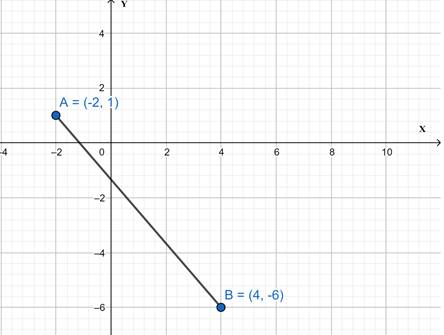# The function.### Precalculus: Mathematics for Calcu...

6th Edition
Stewart + 5 others
Publisher: Cengage Learning
ISBN: 9780840068071### Precalculus: Mathematics for Calcu...

6th Edition
Stewart + 5 others
Publisher: Cengage Learning
ISBN: 9780840068071

#### Solutions

Chapter 2.2, Problem 75E
To determine

## To find : The function.

Expert Solution

The function is y=7x643 .

### Explanation of Solution

Given information : The given coordinates are (2,1) and (4,6) .

Concept used : we must find out the slope of the equation. We can do this by the slope formula for the slope, m , is found by taking the change in y and a dividing it by the change of x .

Graph :Calculation :

m=y2y1x2x1=614+2=76

So,

yy1=m(xx1)y1=76(x+2)y1=7x673y=7x6+173y=7x6+373y=7x643

### Have a homework question?

Subscribe to bartleby learn! Ask subject matter experts 30 homework questions each month. Plus, you’ll have access to millions of step-by-step textbook answers!